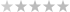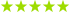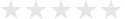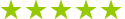# Algebra lessons in Luxembourg

## 0 algebra teachers in Luxembourg

0 teachers in my wish list

## 0 algebra teachers in Luxembourg

Hmm... We couldn’t find any results matching your search. Try online lessons with the following real-time online teachers:(2 reviews)
Asiya - Delft, Netherlands20€
Algebra · Math(12 reviews)
Houssem Eddine - Charleroi, Belgium16€
Math · Algebra(1 review)
Gajanan - India21€
Trusted teacher: I have a background in Master of Technology (Biotechnology) and Bachelor of Pharmacy. I have full time mentor and tutoring since 2004. I have taught AP Calculus AB and BC for the past 17 years. I have taught online math to middle school and high school math course and also teach the college and university level. I have also worked with several college students taking College Algebra, Statistics, Calculus I, and Calculus II, Pre Algebra, Algebra 1, Geometry, Algebra 2, College Algebra, PreCalculus, AP Calculus AB and BC, AP statistics, IB Mathematics: (AA HL SL) Analysis and Approaches HL SL, IB Mathematics: (AI HL SL) Applications and Interpretation HL SL ,The IB Extended Essays (EE), The IB Internal Assessment (IA), Calculus 1, 2 and 3. I have a flexible schedule, and look forward to helping you or your child succeed in math! I also tutor and run summer camps to students succeed on the PSAT (Math), SAT (Math) and ACT (Math ) tests, AMC including helping some students score a perfect score and achieving National Merit Status. In the past year, many of my students have scored 790 or 800 on SAT Math! I also tutor K-12 mathematics, including: FAMAT, MCAT, PSAT, SAT (Science), SSAT, SCAT (School and College Ability Test), PAT, DAT, Canadian DAT, UK A Level & IAL EDXCEL, AQA, OCR, OCR-MEI, WJEC : Core 1, Core 2, Core 3, Core 4, Mechanics 1, Mechanics 2, Mechanics 3, Mechanics 3, Mechanics 4, Mechanics 5, Statistics 1, Statistics 2, Statistics 3, Statistics 4, Further Pure 1,Further Pure 2, Further Pure 3, Decision 1, Decision 2. European Baccalaureate (EB) maths, VCE (Math Method unit 1 and Unit 2), HPAT, GAMSAT (Section 1, 2, and 3), GRE (Math), GMAT (Math), Math Honors, UCAT AP Statistics,(Math 0220, Math 0010, Math 0020, Math 0031, Math 0032, Math 0120, Math 180, Math 190, Math 0200, Math 202, Math 0230, Math 0240, Math 0240, Math 0280, Math 0290, Math 0400) I believe that students learned best when they see the connection of the concept in real life. Also, students master concepts if they have been exposed to the concept in depth. Through practice and systematic ways of solving, all students will be better in Mathematics. I am also willing to take a look at and help with problems and topics not explicitly mentioned above! I look forward to helping you understand math. My ultimate goal is to put myself out of business by making it possible for my students to continue learning without my help. Please contact me to learn more about my schedule. I look forward to working with you!
Test prep · Geometry · Algebra(5 reviews)
Roland - Brussels, Belgium26€
Cpa test preparation · Algebra · Statistics(1 review)
Ilias - Brussels, Belgium32€
Trusted teacher: As a teaching professional with over 17 years’ experience in primary and secondary schools, I aim for the development of independent learners by focusing on inquiry-based student-centred learning. There is a high degree of differentiation in my lessons and activities in order to best meet the educational needs of diverse learners. As a private tutor, I offer support at primary and secondary school level in ICT, Computing and maths. I design activities for the development of creative, analytical and critical thinking while always taking into account the individual learning needs of every student. My Maths support focuses in areas such as: PRIMARY SCHOOL: - WHOLE NUMBERS (operations, comparisons, factors, multiples) - FRACTIONS - MEASUREMENT (Length, mass and volume) - DECIMALS - DATA ANALYSIS (bar graphs, tables) - GEOMETRY (parallel and perpendicular lines, angles, square, parallelograms) - ALGEBRA (algebraic expressions with one variable) SECONDARY SCHOOL - ALGEBRA - Algebraic representation and formulae - translation of simple real-world situations into algebraic expressions - recognising and representing number patterns (including finding an algebraic expression for the nth term) - addition and subtraction of linear algebraic expressions - simplification of linear algebraic expressions, - factorisation of linear algebraic expressions of the form - FUNCTIONS AND GRAPHS - cartesian coordinates in two dimensions • graph of a set of ordered pairs • linear relationships between two variables (linear functions) • the gradient of a linear graph as the ratio of the vertical change to the horizontal change (positive and negative gradients) EQUATIONS AND INEQUALITIES • solving linear equations in one unknown (including fractional coefficients) • solving simple inequality • solving simple fractional equations that can be reduced to linear equations • formulating a linear equation in one unknown to solve problems - GEOMETRY (angles, triangles, circles and polygons) - TRIGONOMETRY - STATISTICS AND PROBABILITIES - 2-D VECTORS - COMPLEX NUMBERS - POLYNOMIALS - DIFFERENTIATION AND INTEGRATION Please do not hesitate to contact me for any further information.
Geometry · Algebra · Math(1 review)
Safiqul - Ongkharak, Thailand16€
Trusted teacher: I have a Doctorate degree in Mathematics. Maths is a wonderful subject, which one needs in all spheres. It is the basic of all subjects. A few applications are defined below: Trigonometry: Calculus is related with trigonometry and algebra. The fundamental trigonometric functions like sine and cosine are used to define the sound and light waves.Trigonometry is further used in oceanography to measure the heights of waves and tides in oceans. It is used in satellite systems, and to create maps. The famous Pythagoras' Theorem finds its' application in determining how tall a ladder needs to be in order to safely place the base away from the wall so it does not turn over. Probability and Statistics: Probability problems infer conclusions about characteristics of hypothetical data taken from the population. Probability explains the mathematical chance that something might happen, and is used in numerous day- to-day applications. Calculus : We can find the (or global) minimum and maximum values of a function as well as the relative (or local) minimum and maximum values of a function. Differential Equation: Differential equations are applied to predict the world around us, and used in a wide variety of disciplines, from biology, economics, physics, chemistry and engineering. It has applications in exponential growth and decay model, Newton's law of cooling, and Circuit theory, e.t.c. I have teaching experience for more than 15 years. My students are from all corners of the globe, and of all ages. I also make them learn Mathematics in English, so that they can excel in future. I am well acquainted will all the topics of mathematics, at all levels from schools to colleges. Children like my style of teaching.
Math · Calculus · Algebra(2 reviews)
Eli - Helsinki, Finland20€
Calculus · Algebra · Math(1 review)
Camille - Chaumont-Gistoux, Belgium18€
Trigonometry · Algebra · Math(2 reviews)
Mohamed - Cairo, Egypt29€
Statistics · Geometry · Algebra
Showing results 1 - 25 of 3861 - 25 of 386

## Our students from Luxembourg evaluate their Algebra teacher.

### To ensure the quality of our Algebra teachers, we ask our students from Luxembourg to review them.Only reviews of students are published and they are guaranteed by Apprentus.Rated 4.7 out of 5 based on 27 reviews.

Math,Calculus,Algebra,Geometry,Arithmetic,Trigonometry,Differential Equations,Mathematical Modeling,Numerical Analysis,Probability and Stats (Nakhon Nayok)
SafiqulMy son really enjoyed his first lesson with Safiqul. He told me that his brain felt 'super good' after the lesson. Just what my son needed - some challenge, kindness and patience. This is what Safiqul provided my son for his maths class. Thank very much and we hope to learn from you again very soon!
Review by ELLY
Math tutoring in English. Let's make math fun and easy! (Helsinki)
EliEli is patient and excellent tutor for my 8th grader son in math. She writes notes each session and emails them to us, which is very helpful. Can strongly recommend her.
Review by HEIDI
Games, animations and interactive stories with Scratch (children and teenagers) (Ixelles-Elsene)
IliasFantastic! Ilias really prepared well and took the time to understand what my son needed. He was very positive and encouraging and we look forward to the next lesson!
Review by SHANNON BELL